ICSE Class 9 Maths Sample Question Paper 8 with Answers

Section – A [40-Marks]
(Attempt all questions from this Section)

Question 1.
(a) The mean of 100 observations was found to be 30. If two observations were wrongly taken as 32 and 12 instead of 23 and 11, find the correct mean.
Here, n = 100, $$\bar{x}$$= 30
∴ Incorrect = Σx= $$\bar{x}$$ n = 30 x 100 = 3000.
∴ Correct Σ x = 3000 – (32 + 12) + (23 + 11)
= 3000 – 44 + 34 = 2990
∴ Correct mean = $$\frac{2990}{100}=29.9$$(b) Determine the rate of interest for a sum that becomes $$\frac{216}{125}$$ times of itself in 3 years, compounded annually.
Let principal be ? P and rate of interest be r% p. a. So,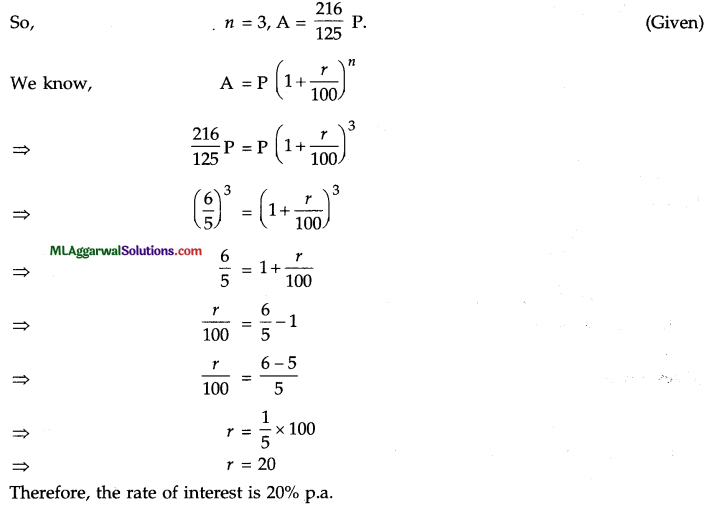(c) Without using tables, find the value of :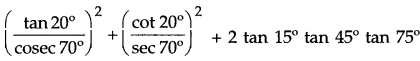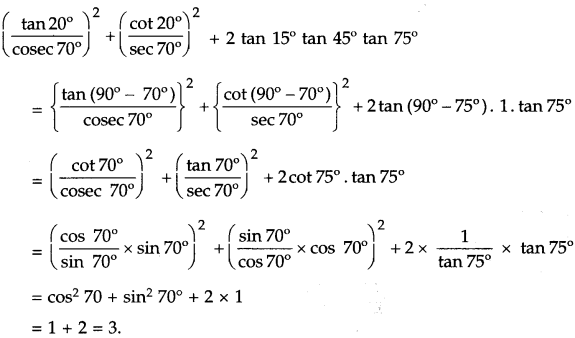Question 2.
(a) If $$x=\frac{3+\sqrt{7}}{2}$$ find the value of $$4 x^{2}+\frac{1}{x^{2}}$$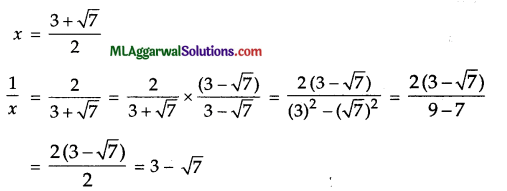(b) In the given figure, ABCD is a rectangle with sides AB = 8 cm and AD = 5 cm. Compute : (i) area of parallelogram ABEF, (ii) area of ΔEFG.Given : AB 8 cm, AD = 5 cm.
(i) Area of parallelogram ABEF = Area of rectangle ABCD
(∵ they are on same base and between same parallels)
= (8 x 5) cm2 = 40 cm2
Area of ΔEFG = $$\frac{1}{2}$$ x Area of parallelogram ABEF
(∵ both are on same base and between same parallels)
= $$\frac{1}{2}$$ x 40 cm2 20 cm2

(c) Without using tables, find the value of :
$$\frac{(b+c)^{2}}{b c}+\frac{(c+a)^{2}}{c a}+\frac{(a+b)^{2}}{a b}$$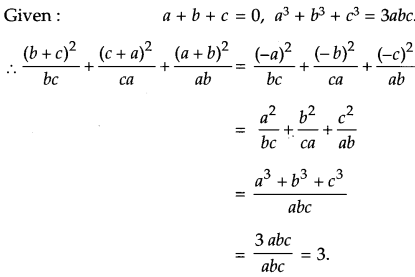Question 3.
(a) Solve for x : 2x +3 + 2x+1 = 320.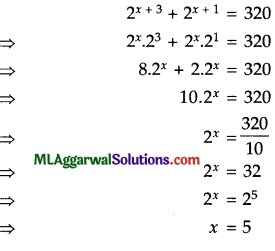(b) In the given figure, AB and CD are two parallel chords and O is the centre. If the radius of the circle is 15 cm, find the distance MN between the two chords of lengths 24 cm and 18 cm respectively.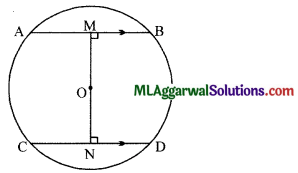Given: radius = OA = OC = 15 cm, AB || CD.
Let AB = 24 cm, CD = 18 cm.
We know perpendicular drawn from centre to the chord, bisects the chord
∴ M and N are mid-point of sides AB and CD respectively.
AM= $$\frac{1}{2}$$ AB= $$\frac{1}{2}$$ x24=12cm.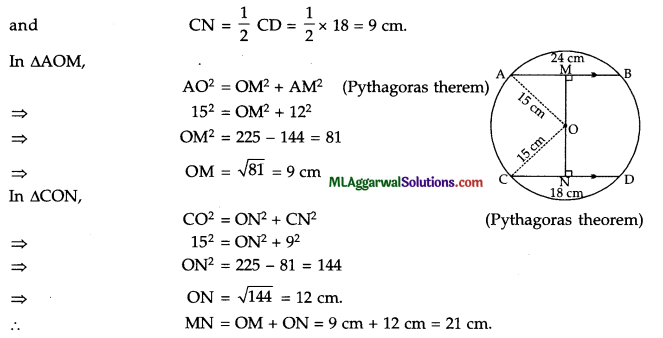(c) Factorize : 4 (2a – 3)2 – 3 (2a – 3) (a – 1) – 7 (a – 1)2.
Given expression is, 4 (2a – 3)2 – 3 (2a – 3) (a – 1) – 7 (a – 1)2
Let 2a – 3 = x and a – 1 = y
The expression becomes
= 4a2 – 3xy – 7y2 = 4x2 – (7 – 4) xy – 7y2
= 4x2 – 7xy + 4xy – 7y2
= x (4x – 7y) + y (4x – 7y)
= (4x – 7y) (x + y)
Substituting values of x and y, we have
= {4 (2a – 3) – 7 (a – 1)} {2a – 3 + a – 1)
= (8a -12 -7a + 7) (3a – 4) = (a – 5) (3a – 4).

Question 4.
(a) Solve for x : log (x + 5) + log (x – 5) = 4 log 2 + 2 log 3.
log {x + 5) + log {x – 5)
=4 log 2 + 2 log 3 log (x + 5) + log (x – 5)
= log 24 + log 32 log (x + 5) + log (x – 5)
= log 16 + log 9 log [(x + 5) (x – 5)]
= log (16 x 9) log(x2 – 25) – log 144
⇒ x2 – 25 = 144
⇒ x2 = 144 + 25 = 169
⇒ x = √169 = 13

(b) Solve simultaneously : $$2 x+\frac{x-y}{6}=2 ; x-\frac{(2 x+y)}{3}=1$$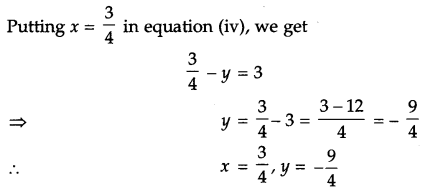(c) If 8 cot 915, find the value of: $$\frac{(2+2 \sin \theta)(1-\sin \theta)}{(1+\cos \theta)(2-2 \cos \theta)}$$Question 5.
(a) The cost of enclosing a rectangular garden with a fence all around at the rate of ₹ 15 per metre is ₹ 5400. If the length of the garden is 100 m, find the area of the garden.
Total cost of fendng = ₹ 5400
Rate = ₹ 15 per metre
Perimeter = $$\frac{5400}{15}$$ = 360 m
Length, l= 100 m
2 (l + b) = 360
b = 180 $$\frac{360}{2}$$ 100 = 80 m
Area = i x b = 100 m x 80 m = 8000 m2

(b) If 4 sin2 x° – 3 = 0 and x° is an acute angle, find (i) sin x° (ii) x°.
Given: 4 sin2 x° – 3 = O
(i) 4 sin2 x° =3
sin2 x° = $$\frac{3}{4}$$
sin x°= $$\frac{\sqrt{3}}{2}$$

(ii) Now sin x°= $$\frac{\sqrt{3}}{2}$$
⇒ sin x°= sin 60°
⇒ x° = 60°(c) Draw a frequency polygon from the following data :

 Age (in years) 25-30 30-35 35-40 40-45 45-50 No. of doctors 40 60 50 35 20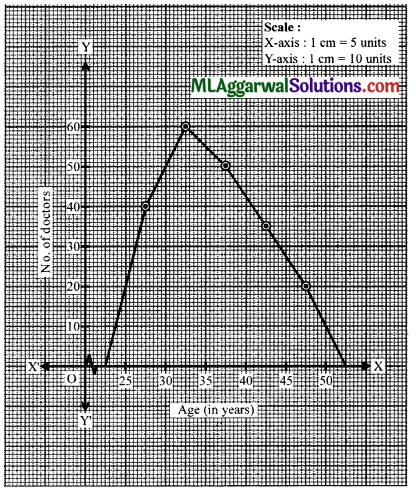Question 6.
(a) If $$\frac{(x-\sqrt{24})(\sqrt{75}+\sqrt{50})}{\sqrt{75}-\sqrt{50}}=1$$ find the value of x.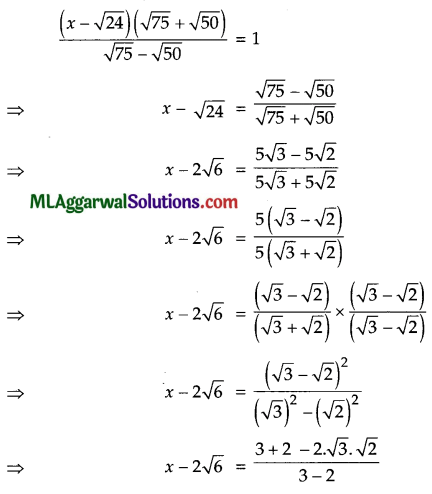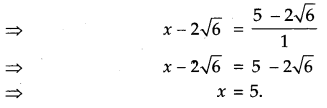(b) From the given figure, find the values of a and b.∠DBC = ∠ADB = a° (Alternate angles)
Now, a + 28° = 75° (Exterior angle is equal to sum of interior opposite angles)
⇒ a = 75° – 28° = 47°.
Also, ∠ABC + ∠BAD = 180° (Co-interior angles)
⇒ a + b + 90° = 180°
⇒ 47° + b + 90° = 180°
⇒ b = 180° – 137° = 43°
a = 47°, b = 43°

(c) Show that the points A (2, – 2), B (8, 4), C (5, 7) and D (- 1, 1) are the vertices of a
rectangle. Also, find the area of the rectangle.i.e., opposite sides are equal and diagonals are equal
ABCD is a rectangle.
Hence Proved.
Area of rectangle = AB x BC = 6√2 x 3√2 = 36 sq. units.

Question 7.
(a) By using suitable identity, evaluate : (9.8).
(9.8)1 = (10 – 0.2)3 = 103 – 3 x 102 x 0.2 + 3 x 10 x (0.2)2 – (0.2)3
= 1000 – 60 + 1.2 – 0.008 = 941.192.

(b) ABC is a triangle right angled at C. A line through the mid-point M of hypotenuse AB
and parallel to BC intersects AC at D. Show that (i) D is mid-point of AC (ii) MD L AC
(iii) CM = MA = $$\frac{1}{2}$$ AB.
Given : M is mid-point of AB, ∠C = 90°, MD||BC.
Join MC.
(i) ∵ M is mid-point of AB and MD|| BC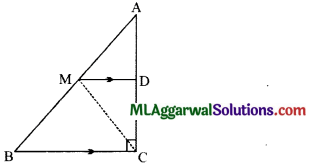∴ By the converse of mid-point theorem,
D is mid-point of AC.
Hence Proved.(ii) ∠BCD + ∠CDM = 1800 (Co-interior angles, MDIIBC)
⇒ 90° + ∠CDM = 180° (∠BCD = 90°)
∠CDM= 180°-90°=90°
MD ⊥ AC. Hence Proved.

(iii) In ΔAMD and ΔCMD,
AD = CD (D is mid-point of AC)
∠ADM = ∠CDM (Each being 90°)
MD = MD (Common side)
∴ ΔAMD ≅ ΔCMD. (SAS axiom)
∴ AM = CM (c.p.c.t.)
Also, AM = $$\frac{1}{2}$$ AB ( M is mid-point of AB)
∴ CM = AM = $$\frac{1}{2}$$ AB.  Hence Proved.

(c) Solve $$x+\frac{1}{x}=2 \frac{1}{2}$$Question 8.
(a) If : a = b2x, b – c2y and c = a2z, show that 8xyz = 1.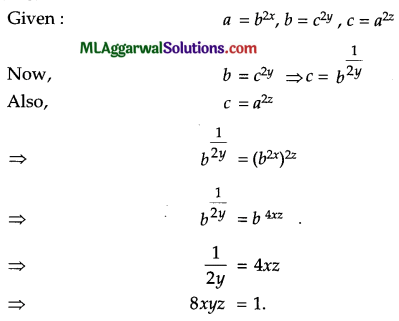(b) In the given figure, ABC is a right triangle at C. If D is the mid-point of BC, prove that AB2 = 4AD2 – 3AC2.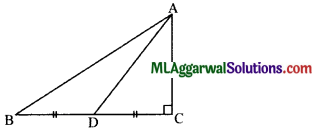Given :∠C = 90°, D is mid-point of BC.
In ΔABC, In ΔACD, ⇒ AB2 = AC2 + BC2 AD2
⇒ AC2 + CD2 CD2
⇒ AD2 – AC2 (Pythagoras theorem) … (i) (Pythagoras theorem)
⇒ $$\left(\frac{1}{2} \mathrm{BC}\right)^{2}$$ = AD2 – AC2 (∵ D is mid-point of BC)
⇒ $$\frac{1}{4}$$ BC2 = AD2 – AC2 4
⇒ BC2 = 4AD2 – 4AC2 …(h)
Using (i) and (ii), we have
AB2 = AC2 + 4AD2 – 4AC2
⇒ AB2 = 4AD2 – 3AC2
Hence Proved.

(c) Find the value of x, if tan 3x = sin 45° cos 45° + sin 30°.
Given: tan 3x = sin 45° cos 45° + sin 30°
⇒  tan 3x $$=\frac{1}{\sqrt{2}} \times \frac{1}{\sqrt{2}}+\frac{1}{2}$$
⇒ tan 3x = $$=\frac{1}{2}+\frac{1}{2}$$
⇒ tan 3x = 1
⇒ tan 3x = tan 45°
⇒ 3x = 45° ,
⇒ 45°
x = $$\frac{45^{\circ}}{3}$$ = 15°Question 9.
(a) Factorize : 12 – (x + x1) (8 – x – x2).
12 – (x + x2) (8 – x – x2) = 12 – (x + x2) {8 – (x + x2)}
Let  x + x2 = a
⇒ 12 – a (8 – a) = 12 – 8a + a2
⇒ a2 – 8a + 12
⇒ a2 – (6 + 2) a + 12
= a2 – 6a – 2m + 12
= a (a – 6) – 2 (a – 6)
⇒ (a-6) (a- 2)
Substituting a = x + x2, we get
⇒ (x + x2 – 6) (x + x2 – 2)
⇒ (x2 + x – 6) (x2 + x – 2)
⇒ (x2 + 3x – 2x – 6) (x2 + 2x – x – 2)
⇒ {x (x + 3) – 2 (x + 3)} {x (x + 2) – 1 (x + 2)}
⇒ (x + 3) (x – 2) (x + 2) (x – 1)
⇒ (x – 1) (x + 2) (x – 2) (x + 3)

(b) A man travels 370 km partly by train and partly by car. If he covers 250 km by train and the rest by car, it takes him 4 hours. But, if he travels 130 km by train and the rest by car, he takes 18 minutes longer. Find the speed of the train and that of the car.
Let the speed of the train be x km/h and that of the car be y km/h.
Total distance travelled = 370 km.
Case I : Distance travelled by train = 250 km.
Distance travelled by car = (370 – 250) km = 120 km
∴ Time taken by train $$=\frac{250}{x} \mathrm{~h}$$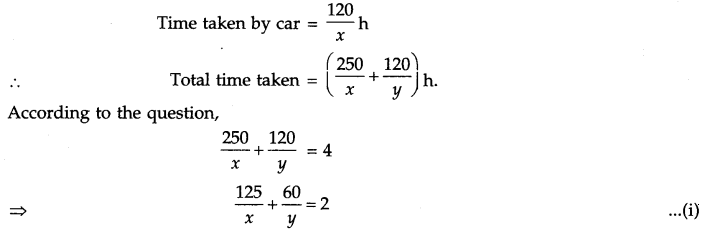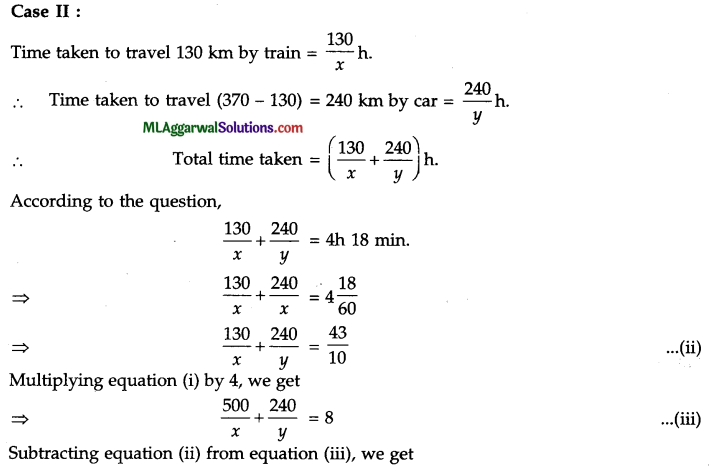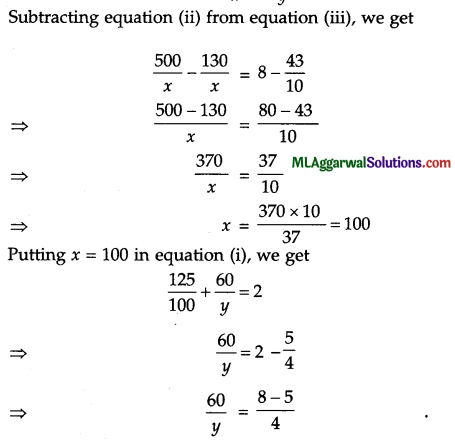(c) Express as a single logarithm :
2 log10 5 – log10 2 + 3log10 4+1
2 log10 5 – log10 2 + 3 log10 4 + 1 = log10 52 – log10 2 + log10 43 + log10 10
$$=\log _{10}\left(\frac{5^{2} \times 4^{3} \times 10}{2}\right)$$
= log10 8000 = log10 (20)3 = 3 log10 20

Question 10.
(a) The value of a car purchased 2 years ago depreciates by 10% every year. Its present value is ₹ 1,21,500. Find the cost price of the car. What will be its value after 2 years ?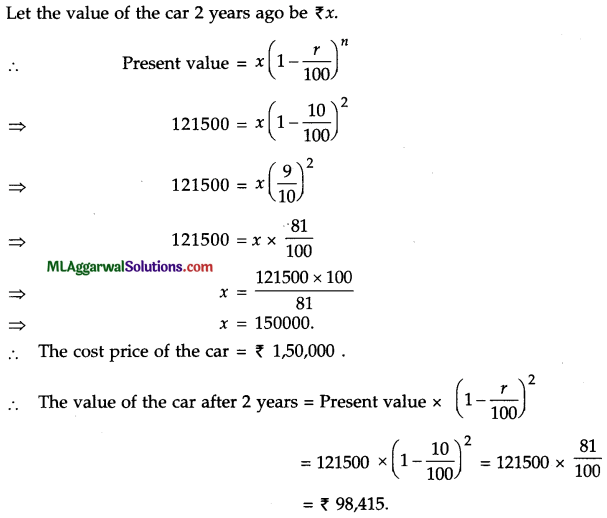(b) Construct a quadrilateral ABCD given that AB = 4.5 cm, ∠BAD = 60°, ∠ABC = 105°, AC = 6.5 cm and AD = 5 cm.
Given : AB 4.5 cm, Z BAD = 60°, Z ABC 105°, AC = 6.5 cm and AD – 5 cm. Steps of
construction :
(1) Draw AB = 4.5 cm.
(2) At A, draw ∠BAX = 60°.
(3) At B, draw ∠ABY = 105°.(4) From A, cut BY at C such that AC = 6.5 cm.
(5) From A, cut AX at D such that AD = 5 cm.
(6) Join CD.
Hence, ABCD is the required quadrilateral.

(c) Factorize : x9 + y9.
= x9 + y9 = (x3)3 + (y3)3 = (x3 + y3) {(x3)2 – x3y3 + (y3)2}
= (x + y) (x2 – xy + y2) (x6 – x3y3 + y6).

Question 11.
(a) In the given figure, ABCD is a parallelogram. Find the values of x, y and z.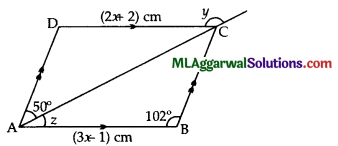Given : ABCD is a parallelogram.
AB = CD
⇒ 3x – 1 = 2x + 2
⇒ 3x – 2x =2 + 1
⇒ x = 3
Also, ∠D = ∠B = 102° ( ∵ Opposite angles are equal) Exterior
In ΔACD, y = 50° + 102° (∵ angle is equal to sum of interior opposite angles)
= 152°
and ∠A + ∠D = 180°
⇒ z + 50° + 102° = 180°
⇒ z = 180° – 152° = 28°
⇒ x = 3, y = 152° z = 28°(b) Draw the graph of 3x + 2 = 0 and 2y – 1 = 0 on the same graph sheet. Do these lines intersect ? If yes, find the point of intersection.
Given: 3x+2=0 ……..(i)
and 2y – 1 = 0 ……. (ii)
From (i), 3x = – 2
= $$x=\frac{-2}{3}$$
It is a straight line parallel to Y-axis at $$x=\frac{-2}{3}$$
From (ii), 2y = 1
⇒ y = $$\frac{1}{2}$$
It is a straight line parallel to Y-axis at y = $$\frac{1}{2}$$(c) Prove that (sin A + cos A)2 + (sin A – cos A)2 = 2.